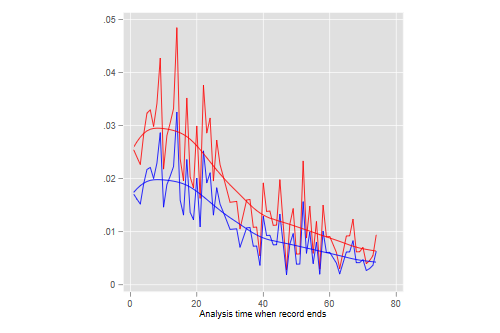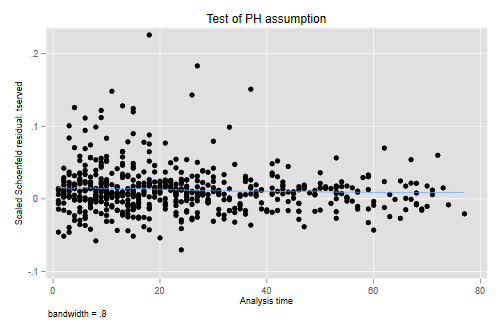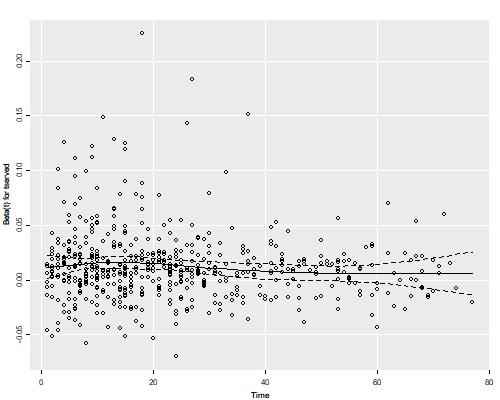Germán Rodríguez
Survival Analysis Princeton University## Cox Proportional Hazards Regression

We return to the recidivism data that we analyzed using parametric models. Let us start by reading the data and setting them up for survival analysis

```. use https://www.stata.com/data/jwooldridge/eacsap/recid, clear

. gen fail = 1 - cens

. stset durat, failure(fail)

Survival-time data settings

Failure event: fail!=0 & fail<.
Observed time interval: (0, durat]
Exit on or before: failure

──────────────────────────────────────────────────────────────────────────
1,445  total observations
0  exclusions
──────────────────────────────────────────────────────────────────────────
1,445  observations remaining, representing
552  failures in single-record/single-failure data
80,013  total analysis time at risk and under observation
At risk from t =         0
Earliest observed entry t =         0
Last observed exit t =        81
```
```> library(haven)
> recid\$fail = 1 - recid\$cens
```

### A Proportional Hazards Model

We fit a proportional hazards model using the efron method for handling ties:

```. local model workprg priors tserved felon drugs black married educ age

. stcox `model', efron

Failure _d: fail
Analysis time _t: durat

Iteration 0:   log likelihood = -3891.7741
Iteration 1:   log likelihood = -3841.8477
Iteration 2:   log likelihood = -3822.6663
Iteration 3:   log likelihood = -3820.9451
Iteration 4:   log likelihood = -3820.9142
Iteration 5:   log likelihood = -3820.9142
Refining estimates:
Iteration 0:   log likelihood = -3820.9142

Cox regression with Efron method for ties

No. of subjects =  1,445                                Number of obs =  1,445
No. of failures =    552
Time at risk    = 80,013
LR chi2(9)    = 141.72
Log likelihood = -3820.9142                             Prob > chi2   = 0.0000

─────────────┬────────────────────────────────────────────────────────────────
_t │ Haz. ratio   Std. err.      z    P>|z|     [95% conf. interval]
─────────────┼────────────────────────────────────────────────────────────────
workprg │   1.054341   .0952997     0.59   0.558     .8831665    1.258691
priors │   1.100222    .014745     7.13   0.000     1.071699    1.129505
tserved │   1.012483   .0016826     7.47   0.000     1.009191    1.015786
felon │   .7361351    .077207    -2.92   0.003     .5993523     .904134
drugs │    1.30457   .1278366     2.71   0.007     1.076607    1.580803
black │   1.485848   .1301791     4.52   0.000     1.251406     1.76421
married │   .8741874   .0953226    -1.23   0.218     .7059735    1.082482
educ │   .9804111   .0190581    -1.02   0.309     .9437606    1.018485
age │   .9966611   .0005162    -6.46   0.000     .9956499    .9976733
─────────────┴────────────────────────────────────────────────────────────────

. estimates store efron // for later comparisons
```
```> library(survival)
> mf <- Surv(durat, fail) ~
+   workprg + priors + tserved + felon + drugs + black + married + educ + age
> cm <- coxph(mf, data = recid)
> cm
Call:
coxph(formula = mf, data = recid)

coef  exp(coef)   se(coef)      z        p
workprg  0.0529156  1.0543406  0.0903879  0.585  0.55826
priors   0.0955123  1.1002224  0.0134018  7.127 1.03e-12
tserved  0.0124059  1.0124832  0.0016618  7.465 8.32e-14
felon   -0.3063417  0.7361351  0.1048815 -2.921  0.00349
drugs    0.2658736  1.3045701  0.0979914  2.713  0.00666
black    0.3959855  1.4858477  0.0876127  4.520 6.19e-06
married -0.1344605  0.8741874  0.1090414 -1.233  0.21753
educ    -0.0197833  0.9804111  0.0194389 -1.018  0.30881
age     -0.0033445  0.9966611  0.0005179 -6.458 1.06e-10

Likelihood ratio test=141.7  on 9 df, p=< 2.2e-16
n= 1445, number of events= 552
```

The estimated coefficients are similar to those obtained using a Weibull model. Here’s a side by side comparison. Recall that R uses the AFT metric, so we need to convert to PH to compare.

```. quietly streg `model', distribution(weibull)

. estimates store weibull

. estimates table efron weibull, equation(1:1) // match equations

─────────────┬──────────────────────────
Variable │   efron       weibull
─────────────┼──────────────────────────
#1           │
workprg │  .05291557    .05775252
priors │   .0955123    .09672029
tserved │  .01240588    .01285382
felon │ -.30634168    -.3201125
drugs │  .26587357    .27186642
black │  .39598547    .41429122
married │ -.13446046   -.12972325
educ │ -.01978331   -.02192692
age │  -.0033445   -.00345801
_cons │              -3.3512677
─────────────┼──────────────────────────
/ln_p │              -.22080169
─────────────┴──────────────────────────
```
```> wm <- survreg(mf, dist = "weibull", data = recid)
> cbind( coef(cm), -coef(wm)[-1]/wm\$scale )
[,1]         [,2]
workprg  0.052915570  0.057752517
priors   0.095512305  0.096720295
tserved  0.012405884  0.012853823
felon   -0.306341680 -0.320112498
drugs    0.265873573  0.271866422
black    0.395985470  0.414291221
married -0.134460460 -0.129723255
educ    -0.019783310 -0.021926923
age     -0.003344497 -0.003458007
```

For example the Cox coefficient for blacks indicates that African Americans face a 48.6% higher risk of recidivism at any duration since release from prison than non-blacks with the same observed characteristics. The Weibull analysis yielded an estimate of 51.3% higher hazard.

### The Treatment of Ties

Let us compare all available methods of handling ties. If you run this code expect the exact calculation to take substantially longer than the others.

```. quietly stcox `model', breslow // default

. estimates store breslow

. quietly stcox `model', exactm

. estimates store exactm

. quietly stcox `model', exactp

. estimates store exactp

. estimates table breslow efron exactm exactp

─────────────┬────────────────────────────────────────────────────
Variable │  breslow       efron        exactm       exactp
─────────────┼────────────────────────────────────────────────────
workprg │  .05284355    .05291557    .05291409    .05315276
priors │  .09503255     .0955123    .09551509    .09645497
tserved │  .01229776    .01240588    .01240713    .01254959
felon │ -.30525144   -.30634168    -.3063558   -.30993357
drugs │  .26393017    .26587357    .26588358    .26686928
black │  .39366079    .39598547    .39600249      .398402
married │ -.13422414   -.13446046   -.13446367   -.13577505
educ │ -.01971757   -.01978331   -.01978529   -.02012032
age │  -.0033222    -.0033445   -.00334456   -.00335309
─────────────┴────────────────────────────────────────────────────
```
```> cm_b <- coxph(mf, data=recid, ties="breslow")
> cm_e <- coxph(mf, data=recid, ties="exact")
> data.frame(breslow = coef(cm_b), efron = coef(cm), exactp = coef(cm_e))
breslow        efron       exactp
workprg  0.052843554  0.052915570  0.053152759
priors   0.095032546  0.095512305  0.096454966
tserved  0.012297764  0.012405884  0.012549587
felon   -0.305251441 -0.306341680 -0.309933573
drugs    0.263930169  0.265873573  0.266869280
black    0.393660789  0.395985470  0.398402001
married -0.134224141 -0.134460460 -0.135775053
educ    -0.019717572 -0.019783310 -0.020120315
age     -0.003322199 -0.003344497 -0.003353089
```

As is often the case, the Efron method comes closer to the exact partial likelihood estimate with substantially less computational effort, although in this application all methods yield very similar results.

### Baseline Hazard

After fitting a Cox model we can obtain estimates of the baseline survival or cumulative hazard using extensions of the Kaplan-Meier and Nelson-Aalen estimators.

Estimates of the hazard itself, which may be obtained by differencing the estimated cumulative hazard or negative the estimated survival, are usually too “spiky” to be useful, but can be smoothed to glean the general shape.

Stata makes these calculations extremely easy via the `stcurve` command. By default the command computes the baseline hazard or survival setting all covariates to their means, not zero. You can request other values via the options `at(varname=value)`. Below I plot the hazards for blacks and others with all other variables set to their means. To make sure you understand exactly what Stata is doing under the hood I also do this “by hand”.

In R we can obtain the survival via `survfit()`, which accepts a Cox model as argument. You are encouraged to always call this function with a new data frame that specifies exactly what you want to calculate. The default is the mean of all covariates used in the Cox fit. Below I calculate the means explicitly and construct a data frame with two rows, representing blacks and others with all other variables set to their means. I then compute the survival, and difference the negative log to obtain the hazard.

```. stcurve, at(black=1) at(black=0) hazard

. // by hand:
. preserve

. quietly sum black

. scalar mb = r(mean)

. predict H, basech

. keep if fail
(893 observations deleted)

. bysort _t: keep if _n == 1
(478 observations deleted)

. gen h= H[_n + 1] - H
(1 missing value generated)

. gen rr0 = exp((1 - mb) * _b[black])

. gen rr1 = exp( - mb    * _b[black])

. gen h0 = h * rr0
(1 missing value generated)

. gen h1 = h * rr1
(1 missing value generated)

. line h0 h1 _t, col(red blue) legend(off)

. lowess h0 _t, gen(s0) bw(0.5) nograph

. lowess h1 _t, gen(s1) bw(0.5) nograph

. line h0 h1 s0 s1 _t, col(red blue red blue) legend(off) aspect(1)

. restore

. graph export recidhaz.png, width(500) replace
file recidhaz.png saved as PNG format
```
```> library(dplyr)
> library(ggplot2)
> means <- summarize_each(recid, funs(mean))
> nd <- rbind(means, means) %>% mutate(black = 0:1)
> sf <- survfit(cm, newdata = nd) # type will match estimate
> nr <- length(sf\$time)
> ndh <- data.frame(
+   ethn = factor(rep(c("black","other"), rep(nr - 1, 2))),
+   time = (sf\$time[-1] + sf\$time[-nr])/2,
+   hazard = c(diff(-log(sf\$surv[,2])), diff(-log(sf\$surv[,1])))
+ )
> ggplot(ndh, aes(time, hazard, color = ethn)) + geom_line() +
+     geom_smooth(span = 0.5, se = FALSE)
> ggsave("recidhazr.png", width = 500/72, height = 400/72, dpi = 72)
```The estimates suggests that the hazard raises a bit in the first few weeks after release and then declines with duration. This result is consistent with the observation that a log-normal model fits better than a Weibull.

### Schoenfeld Residuals

We now check the proportional hazards assumption using scaled Schoenfeld residuals. Recall that our software uses different defaults so results will differ. Stata computes the test using the original time scale. R computes it using the overall survival function as the time scale. We specify `transform = "identity"` to obtain exactly the same results.

```. estimates restore efron
(results efron are active now)

. estat phtest, detail

Test of proportional-hazards assumption

Time function: Analysis time
─────────────┬──────────────────────────────────────────
│        rho     chi2       df    Prob>chi2
─────────────┼──────────────────────────────────────────
workprg │    0.04790     1.27        1       0.2595
priors │   -0.08288     3.06        1       0.0802
tserved │   -0.09808     3.59        1       0.0580
felon │    0.00891     0.04        1       0.8423
drugs │   -0.00486     0.01        1       0.9090
black │    0.04834     1.27        1       0.2591
married │    0.04923     1.38        1       0.2399
educ │   -0.06091     1.83        1       0.1766
age │    0.04229     1.31        1       0.2531
─────────────┼──────────────────────────────────────────
Global test │               12.76        9       0.1740
─────────────┴──────────────────────────────────────────
```
```> zph <- cox.zph(cm, transform = "identity"); zph
chisq df     p
workprg  1.26387  1 0.261
priors   1.28885  1 0.256
tserved  2.96405  1 0.085
felon    0.23298  1 0.629
drugs    0.00763  1 0.930
black    0.82568  1 0.364
married  2.97696  1 0.084
educ     0.81276  1 0.367
age      0.41025  1 0.522
GLOBAL  12.67933  9 0.178
```

The overall chi-squared statistic of 12.76 on 9 d.f. indicates no significant departure from the proportional hazards assumption. The only variable that might deserve further scrutiny is time served, which has the largest chi-squared statistic, although it doesn’t reach the conventional five percent level. Just out of curiosity we can plot the scaled Schoenfeld residuals against time. To do this we use the `plot(varname)` option of `stphtest`.we use the generic `plot()` function, called via `ggfy()` to make the plot look a bit like `ggplot`, with the object `zph` that includes the index of the variable of interest.

```. stphtest, plot(tserved)

. graph export recidpht.png, width(500) replace
file recidpht.png saved as PNG format
```
```> source("ggfy.R.txt")
> png(file="recidphtr.png", width=500, height=400)
> ggfy(zph) # or call plot(zph) directly
> dev.off()
pdf
2
```We see no evidence of a trend in the effect of time served, so we have no evidence against the proportionality assumption.

More detailed exploration of this issue can be pursued by introducing interactions with duration, as we demonstrated using Cox’s example.

